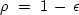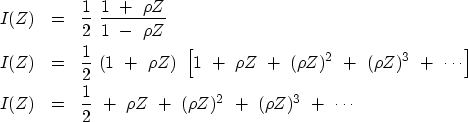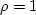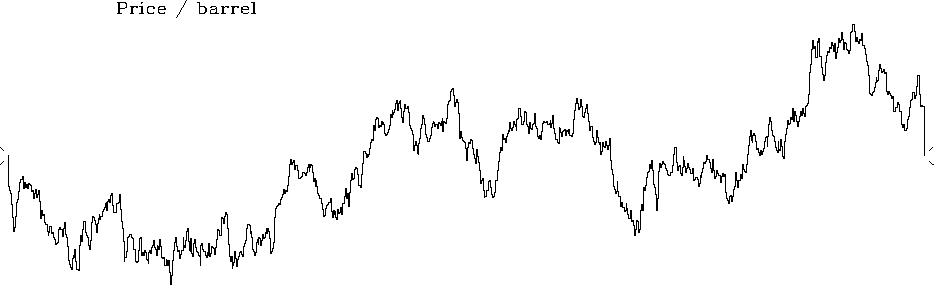Next: Symmetrical double integral Up: CAUSAL INTEGRATION FILTER Previous: The accuracy of causal

## Examples of causal integration

The integration operator has a pole at Z = 1, which is exactly on the unit circle |Z|=1. The implied zero division has paradoxical implications (page) that are easy to avoid by introducing a small positive numberand defining.The integration operator becomes(23) (24)
Becauseis less than one, this series converges for any value of Z on the unit circle. Ifhad been slightly negative instead of positive, a converging expansion could have been carried out in negative powers of Z. A plot of I(Z) is found in Figure 4.cint
Figure 4
A leaky causal-integration operator I.

Just for fun I put random noise into an integrator to see an economic simulation, shown in Figure 5. With, the difference between today's price and tomorrow's price is a random number. Thus the future price cannot be predicted from the past. This curve is called a random walk."price
Figure 5
Random numbers into an integrator.Next: Symmetrical double integral Up: CAUSAL INTEGRATION FILTER Previous: The accuracy of causal
Stanford Exploration Project
10/21/1998